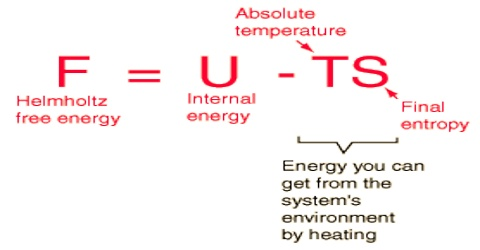Chemistry

# The Free Energy and the Work FunctionThe Free Energy and the Work Function

We have already established the criterion of spontaneous processes and equilibrium. Spontaneous processes, whether chemical or physical, often have:

• A negative internal energy change, (release of heat energy, ∆UV < 0)
• A negative enthalpy change, (release of heat energy, ∆HP < 0)
• An increase in entropy, (increase in disorder, ∆SV > 0)

Thus, it appears that spontaneity of a process involve thermodynamic properties -internal energy, enthalpy and entropy. For practical purposes, however, these criteria are generally inconvenient, since it requires knowledge of the properties of the surroundings in addition to those of the substances of primary interest. This inconvenience has been minimized by the introduction of two more thermodynamic functions which are derived from the functions U. H and S. The functions are:

(i) Free Energy or Gibbs Free Energy, G

William Gibbs, an American mathematical physicist, developed an equation in 1870s, combining enthalpy and entropy contributions. This equation provided a means to describe energy content and therefore, a means to evaluate the spontaneity at a reaction when that energy content changes. The energy content of a substance was termed the Gibbs Free Energy, G.

In the relation: G = H – TS

H is enthalpy, S is entropy and T is the temperature in Kelvin.

(ii) Work Function or Helmholtz Free Energy, A

In 1882 the German physicist and physiologist Hermann von Helmholtz coined the term ‘free energy’ for the function. A which is given by, A = U – TS

U is the internal energy and the other terms have the same significance.

The term ‘free energy’, however, now refers to Gibbs free energy, G. The Helmholtz free energy function, A, is generally used to describe the change in a system at constant temperature and constant volume.